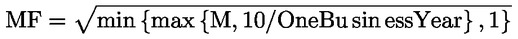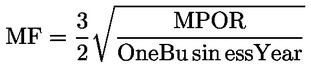In force

Version from: 01/01/2023
Amendments
Search within this legal act

### Article 279c - Maturity Factor

Article 279c

Maturity Factor

1.

Institutions shall calculate the maturity factor as follows:

(a)

for transactions included in the netting sets referred to in Article 275(1), institutions shall use the following formula:where:

 MF = the maturity factor;
 M = the remaining maturity of the transaction which is equal to the period of time needed for the termination of all contractual obligations of the transaction; for that purpose, any optionality of a derivative contract shall be considered to be a contractual obligation; the remaining maturity shall be expressed in years using the relevant business day convention; where a transaction has another derivative contract as underlying instrument that may give rise to additional contractual obligations beyond the contractual obligations of the transaction, the remaining maturity of the transaction shall be equal to the period of time needed for the termination of all contractual obligations of the underlying instrument; where a transaction is structured to settle outstanding exposure following specified payment dates and where the terms are reset so that the market value of the transaction is zero on those specified dates, the remaining maturity of the transaction shall be equal to the time until the next reset date; and
(b)

for transactions included in the netting sets referred to in Article 275(2) and (3), the maturity factor is defined as:where:

 MF = the maturity factor;
 MPOR = the margin period of risk of the netting set determined in accordance with Article 285(2) to (5); and